# IIT JAM Chemistry - MCQ Test 3

## 60 Questions MCQ Test MOCK Test Series for IIT JAM Chemistry | IIT JAM Chemistry - MCQ Test 3

Description
This mock test of IIT JAM Chemistry - MCQ Test 3 for Chemistry helps you for every Chemistry entrance exam. This contains 60 Multiple Choice Questions for Chemistry IIT JAM Chemistry - MCQ Test 3 (mcq) to study with solutions a complete question bank. The solved questions answers in this IIT JAM Chemistry - MCQ Test 3 quiz give you a good mix of easy questions and tough questions. Chemistry students definitely take this IIT JAM Chemistry - MCQ Test 3 exercise for a better result in the exam. You can find other IIT JAM Chemistry - MCQ Test 3 extra questions, long questions & short questions for Chemistry on EduRev as well by searching above.
QUESTION: 1

Solution:
QUESTION: 2

Solution:
QUESTION: 3

### The moment of inertia for HCl gas can be determined from its microwave spectrum, which property of the HCl molecule may be obtained from the moment of inertia:

Solution:
QUESTION: 4

Overtones are observed in the vibrational spectra of diatomic molecules when:

Solution:
QUESTION: 5

The correct order of the fundamental vibrational frequency of the following diatomic molecules is:

Solution:
QUESTION: 6

The reaction of the bromo compound shown below with sodium ethoxide gives predominantly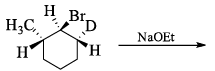Solution:
QUESTION: 7

The reaction of l-bromo-2-fluorobenzene with furan in the presence of one equivalent to Mg gives:

Solution:
QUESTION: 8

Correct absolute configuration is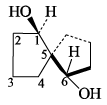Solution:
QUESTION: 9

The microwave spectrum of a molecule yields three rotational constants. The molecule is:

Solution:
QUESTION: 10

Electronegativity and Electron affinity of an element A are X and Y resp. Hence Ionisation potential is

Solution:
QUESTION: 11

Which of the following is the most stable conformer of the following molecule: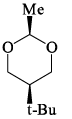Solution:

Explanation : When the methyl group in the structure above occupies an axial position it suffers steric crowding by the two axial hydrogens located on the same side of the ring. The conformation in which the methyl group is equatorial is more stable, and thus the equilibrium lies in this direction.

Therefore,the equatorial bonds are the most stable in chair form.

QUESTION: 12

The increasing order of basicity among the following is :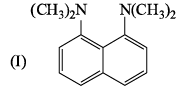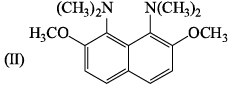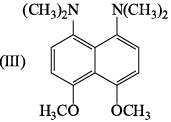Solution:
QUESTION: 13

The major product P is: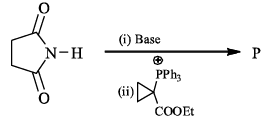Solution:
QUESTION: 14

The major product expected from the following reaction is?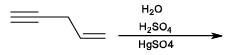Solution:
QUESTION: 15

The population (N) distribution over states (n) of a diatomic molecular corresponds to: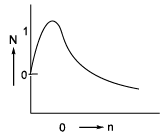Solution:
QUESTION: 16

Arrange the following in increasing order of Ka value: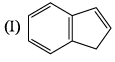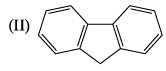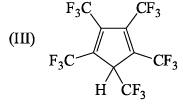Solution:
QUESTION: 17

Correct energy profile for amine inversion and hybridization of nitrogen in transition state is: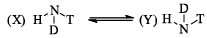Solution:
QUESTION: 18

Resonance frequencies for EPR and NMR are respectively in the spectral region:

Solution:
QUESTION: 19

Compound A and B exist in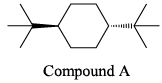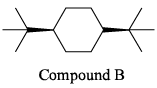Solution:
QUESTION: 20

Consider the cell Cd(s)/Cd+2(1.0M)// C+2(1.0M)/Cu(s). if we wanted to make a cell with a more positive voltage using the same substances, we should:

Solution:
QUESTION: 21

The major product P is: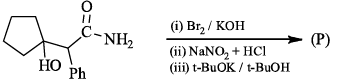Solution:
QUESTION: 22

EMF of the following cell is 0.67 V at 298 K. Pt|H2(latm) |H+(pH = X) || KCl (IN) | Hg2Cl2(s) | Hg Calculate the pH of anode compartment. Given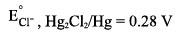Solution:
QUESTION: 23

Rate of formation of SOin the following reaction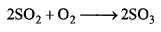is 100g min-1. Hence, rate of disappearance of O2 is:

Solution:
QUESTION: 24

In the following sequence of pericyclic reactions X and Y are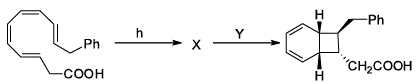Solution: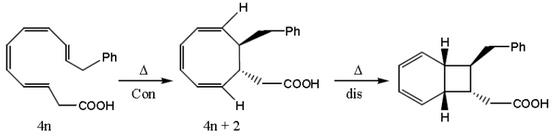QUESTION: 25

Specifi heat of N2 at constant pressure is 0.25 cal/g°C. Hence specific heat at constant volume is

Solution:
QUESTION: 26

Bridge-head hydrogen of the conform of cis-decalin is positioned as:

Solution:
QUESTION: 27

The resonance energy of anthracene (from the below data)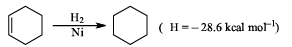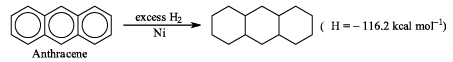Solution:
QUESTION: 28

Given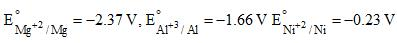which of the following statement is incorrect:

Solution:
QUESTION: 29

Weight of 14C have radioactivity 1 Curie (Disintegration constant is 4.4 × 10–12 sec-1 is ‘(in Kg)

Solution:
QUESTION: 30

In the reaction, P + Q → R + S the time taken for 75% reaction of P is twice the time taken for 50% reaction of P. The concentration of Q varies with reaction time as shown in the figure. The overall order of the reaction is: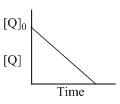Solution:
*Multiple options can be correct
QUESTION: 31

Consider the following reaction involving two acids shown below: formic acid and HF.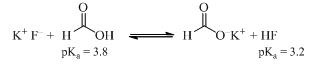Solution:
*Multiple options can be correct
QUESTION: 32

Which statement is correct in respect of the below reaction: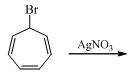Solution:
*Multiple options can be correct
QUESTION: 33

Which of the following statement is/are correct:

(I) The conductance of one cm3 (or 1 unit3) of a solution is called specific conductance

(II) Specific conductance increases while molar conductively decreases on progressive dilution

(III) The limiting equivalent conductivity of weak electrolyte cannot be determine exactly by extrapolation of the plot of ∧eq against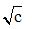(IV) The conductance of metals is due to the movement of free electrons

Solution:
*Multiple options can be correct
QUESTION: 34

In electrolyte concentration cell:

(I) The electrode material and the solution in both half –cells are composed of the same substances

(II) Only the concentrations of solutions of the same substances is different

(III) E0cell = 0

(IV) The Nernst equation reduces to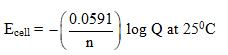Which of the following statement is correct:

Solution:
*Multiple options can be correct
QUESTION: 35

Dimer formation can take place in

Solution:
*Multiple options can be correct
QUESTION: 36

Which of the following complex species is expected to show optical isomerism-

Solution:
*Multiple options can be correct
QUESTION: 37

Boric acid is dissolved in D2O. Hence, which of the following is/are true

Solution:
*Multiple options can be correct
QUESTION: 38

Mg and Zn do not resemble in following properties

Solution:
*Multiple options can be correct
QUESTION: 39

Which of the following compound is/ are chiral: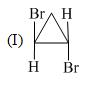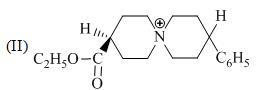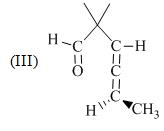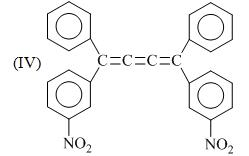Solution:
*Multiple options can be correct
QUESTION: 40

Which among the following is/are acid:

Solution:
*Answer can only contain numeric values
QUESTION: 41

If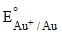is 1.69 V &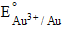is 1.40 V, then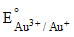will be(in Volt):

Solution:
*Answer can only contain numeric values
QUESTION: 42

Consider the following nuclear reactions: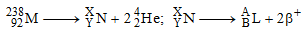The number of neutrons in the element L is:

Solution:
*Answer can only contain numeric values
QUESTION: 43

The two fine-structure components of a nuclear magnetic resonance transition are observed  at chemical shifts of 2.142 and 2.208 ppm in a 300 MHz NMR spectrometer. Calculate the coupling constant (in Hz):

Solution:
*Answer can only contain numeric values
QUESTION: 44

What is the oxidation state of ‘W’ in (Me3CO)3W(CCMe3) ?

Solution:
*Answer can only contain numeric values
QUESTION: 45

B2H6 is added with excess of RCl. Number of the possible products among following:

B2H1R5 ,B2H2R4, B2H3R3 , B2H5R1, B2R6, B2H4R2

Solution:
*Answer can only contain numeric values
QUESTION: 46

Number of molecules among the following, having pЛ-dЛ bonding is/are

P4O10, N2O5, (SiH3)3N, SO3.

Solution:
*Answer can only contain numeric values
QUESTION: 47

How many P-O-P bond are there in H27P25O76  andH25P25O75 resp:

Solution:
*Answer can only contain numeric values
QUESTION: 48

Half life of 53I125 is 60 days. What percentage of radioactivity would be present after 180 days ?

Solution:
*Answer can only contain numeric values
QUESTION: 49

What would be the atomic weight of isotope of Hydrogen which contains 2 neutrons in nucleus.

Solution:
*Answer can only contain numeric values
QUESTION: 50

For the fuel cell reaction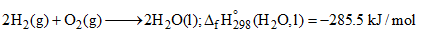What is ΔS0298 (kJ/K) for the given fuel cell reaction?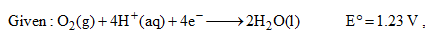Solution:
*Answer can only contain numeric values
QUESTION: 51

Rotational property of a body is characterized by its moment of inertia. Consider ABCD is square box of side 4m. Find moment of inertia (in Kg/m2) along Z-axis: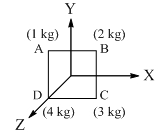Solution:
*Answer can only contain numeric values
QUESTION: 52

Ionisation energy of He+  is 19.6 × 10–18 Jatom-1.The energy of first stationary state of Li+2 is…(in J atom-1 , in the multiple of 10–17):

Solution:
*Answer can only contain numeric values
QUESTION: 53

At 570 C gaseous dinitrogentetraoxide is 50% dissociated. Calculate standard free energy change (J/mol) of it at this temp and 1atm initial pressure of gas:

Solution:
*Answer can only contain numeric values
QUESTION: 54

Equal volume of two monoatomic gases A and B are mixed at same temp. and pressure.  The ratio of specific heats Cp:Cvof mixture will be …?

Solution:
*Answer can only contain numeric values
QUESTION: 55

For the reaction X2O4(l) giving 2XO2(g) , ∆U = 2.1 kcal, ∆S= 20 cal/K at 300K. Calculate ∆G(in kcal):

Solution:
*Answer can only contain numeric values
QUESTION: 56

A sample of rock from moon contains equal number of atoms of Uranium and Lead (t1/2 for
U = 4.5× 109 years. What is age (years) of rock (in the multiple of 109)?

Solution:
*Answer can only contain numeric values
QUESTION: 57

Consider the following reaction,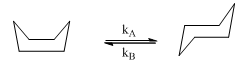The reaction is first order in each direction, with an equilibrium constant of 104. For the conversion of chair form to boat form e–Ea/RT = 4.35 × 10–8 at 298 K with pre-exponential factor of 1012 s–1, Apparent rate constant (=kA + kB) at 298 K is(S–1, in the multiple of 108):

Solution:
*Answer can only contain numeric values
QUESTION: 58

Following is the graph between (a – x)–1 and time t for a second order reaction,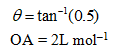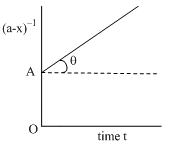Hence, rate at the start of the reaction is (in mol L–1 min–1 ).

Solution:
*Answer can only contain numeric values
QUESTION: 59

The number of neutrons emitted when 92U235 undergoes controlled nuclear fission to 54Xe142 and 38Sr90.

Solution:
*Answer can only contain numeric values
QUESTION: 60

The J = 0 to J = 1 rotational transition for 1H79Br occurs at 500.72 GHz. Assuming the molecule to be a rigid rotor, the J = 3 to J = 4 transition occurs at …….(in cm–1)

Solution: